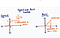# Recap

In the previous posts, I covered the following topics

In the third part of the series, I will cover three functions that will be used during forward propagation.

1. ReLU
2. Maxpooling
3. Softmax

# ReLU Function

The ReLU function is a non-linear activation function which filters out negative values.

ReLU function applied in a neural network does not face problems such as vanishing gradients. ReLU function result in non zero gradients for positive values , unlike saturating functions such as sigmoid.

Given that the differential of a ReLU function is a constant, lesser time is need to compute the gradient of a layer with ReLU activation.Large positive values applied to a sigmoid function will converge to 1. This results in the derivative being zero for large positive values. Zero derivatives will prevent model parameters from adjusting correctly.

The following code snippet contains the function for ReLU

`def ReLU(x):    return (x>0)*x`

# Max pooling

Max pooling is a process to extract low level features in the image. This is done by picking image chunks of pre-determined sizes, and keeping the largest values from each of these chunks.

A single max pool operation results in picking a chunk of image, which has the same size as the max pool filter , and choosing the maximum value from that. Multiple max pool operations are done based on how much we allow a max pool filter to move after each max pool operation. This is decided by a pre-defined parameter called stride.

In this project, I have chosen the stride = 2 and image width & height = 2.

The following diagram shows a simple example of max pool operation applied to an image with just one channel.

The following diagram shows the max pool operation applied to image with two channels. The number of channels do not change. The height / width of the image changes based on the chosen filter height/width

The new height or width is calculated using the following

New Height or New Width = ((Image Height or Width-Filter Height or Width+2*padding)/stride)+1

`def maxpool_multiple(input_image,stride=2):    input_width = input_image.shape    input_height = input_image.shape    filter_width = 2    filter_height = 2        output_width = int((input_width-filter_width)/stride)+1    output_height = int((input_height-filter_height)/stride)+1        output_image = np.zeros((input_image.shape,input_image.shape,output_width,output_height))    for i in range(output_image.shape):        output_image[i:i+1,:,:,:] = maxpool(input_image[i:i+1,:,:,:],stride=2)    return output_imagedef maxpool(input_image,stride=2):    input_width = input_image.shape    input_height = input_image.shape    filter_width = 2    filter_height = 2    n_channels = input_image.shape    num_images = input_image.shape         output_width = int((input_width-filter_width)/stride)+1    output_height = int((input_height-filter_height)/stride)+1    output = np.zeros((n_channels,output_width*output_height))    c=0    for height in range(0,input_height,stride):        if height+filter_height<=input_height:            image_rectangle = input_image[0,:,height:height+filter_height,:]            for width in range(0,input_width,stride):                if width+filter_width<=input_width:                    image_square = image_rectangle[:,:,width:width+filter_width]                    image_flatten = image_square.reshape(-1,1)#                     print(image_flatten)#                     print('----')                    output[:,c:c+1] = np.array([float(max(i)) for i in np.split(image_flatten,n_channels)]).reshape(-1,1)                    c+=1                   final_output = np.array(np.hsplit(output,1)).reshape((1,n_channels,output_height,output_width))            return final_output`

# Soft max Function

The softmax function converts a vector of real values to a vector of values that range between 0 to 1. The newly transformed vector adds up to 1; the transformed vector becomes a probability distribution. A large value will be transformed to a value that is close to 1, a small value will be transformed to a value that is close to 0.

The soft max function will be used at the last layer for prediction; if the 1st node has the highest value, the prediction will be 0. If the 3rd node has the highest value, the prediction will be 2.

In the following example, we will look at the softmax operation.

The softmax transformation will look like the following way

# Feedback

Thank You for reading ! If you have any feedback/suggestions, please feel free to comment below/ you can email me at padhokshaja@gmail.com

# Next Post

Back propagation through fully connected layers

## Analytics Vidhya

Analytics Vidhya is a community of Analytics and Data…

## Analytics Vidhya

Analytics Vidhya is a community of Analytics and Data Science professionals. We are building the next-gen data science ecosystem https://www.analyticsvidhya.com

Written by